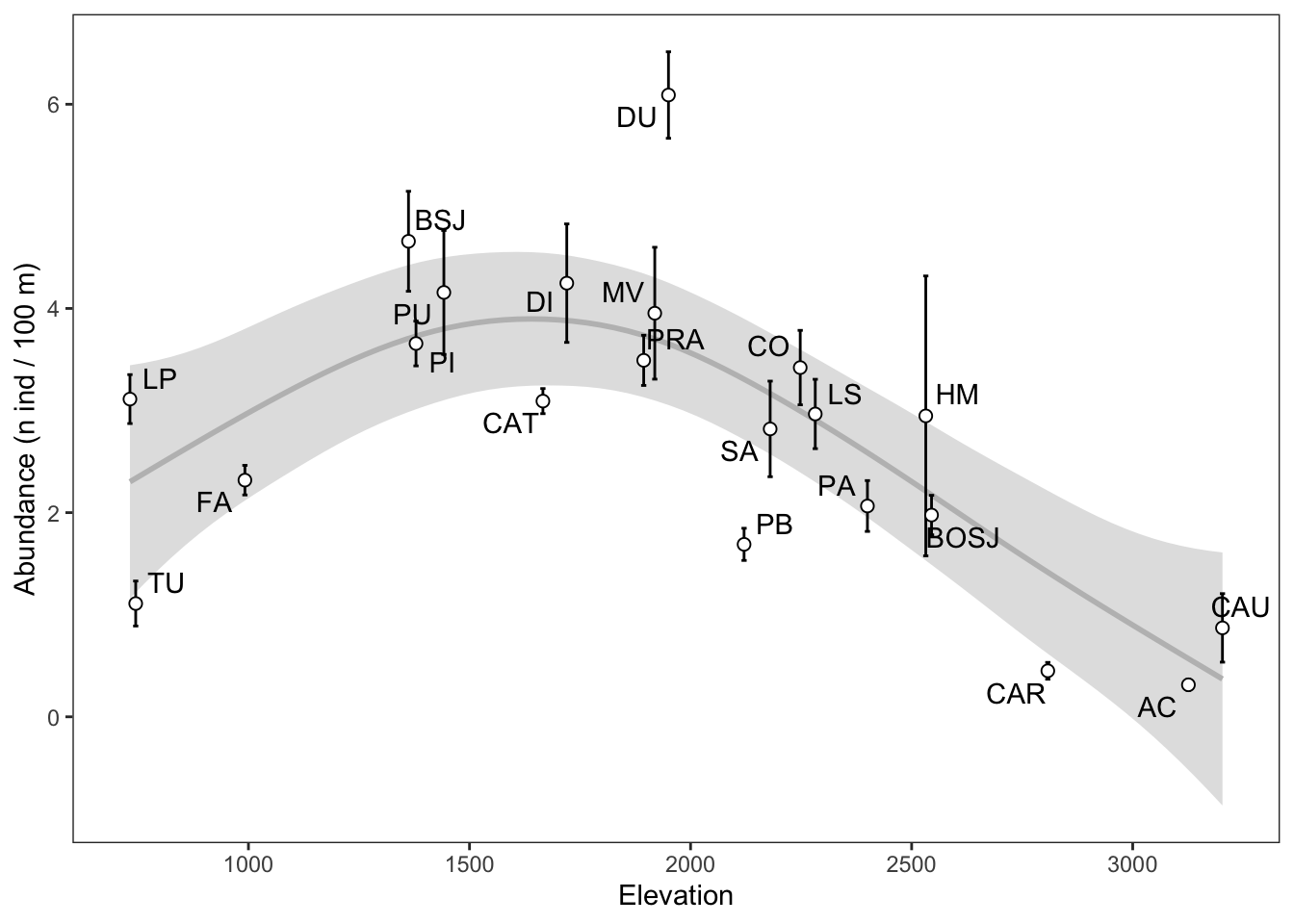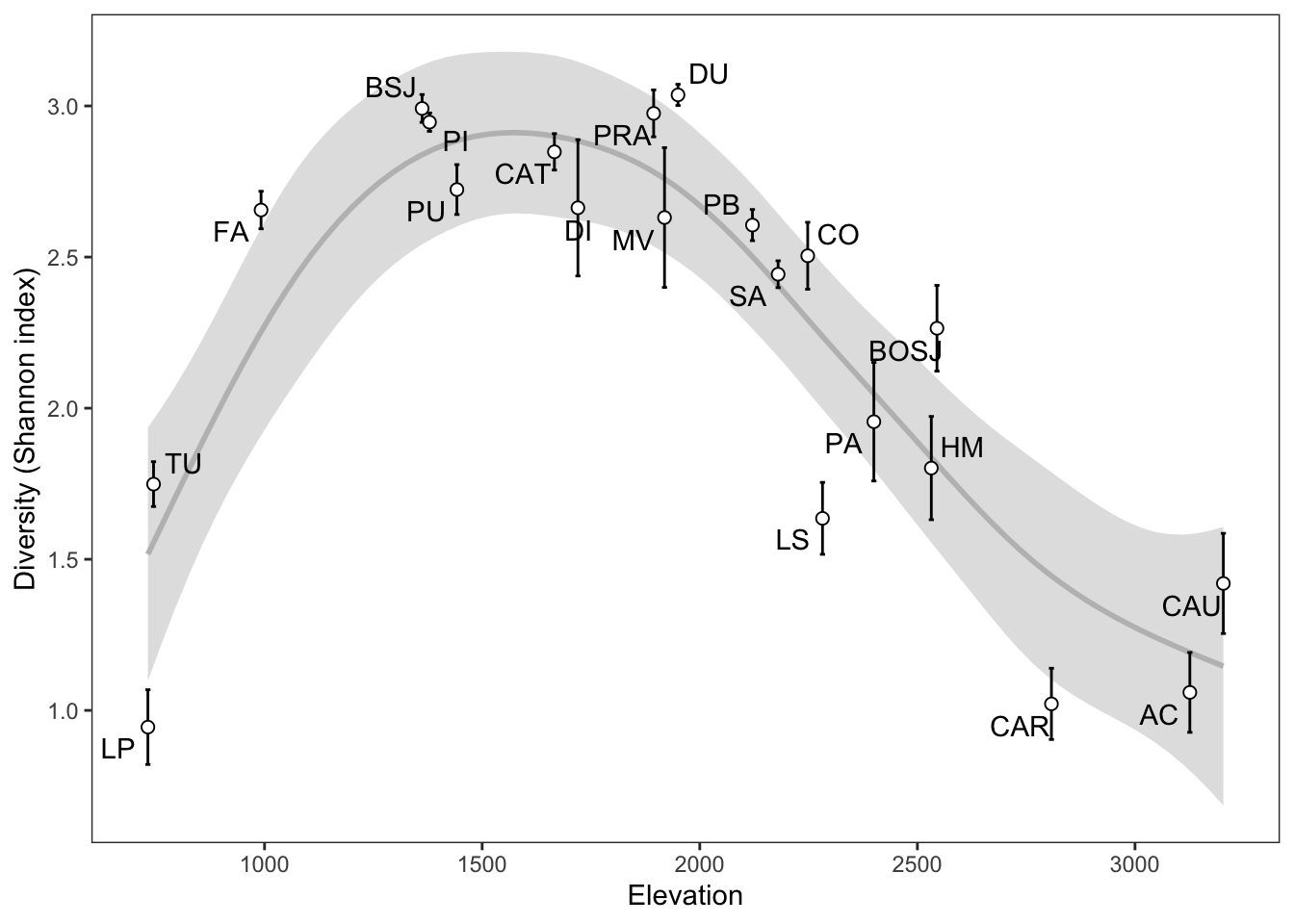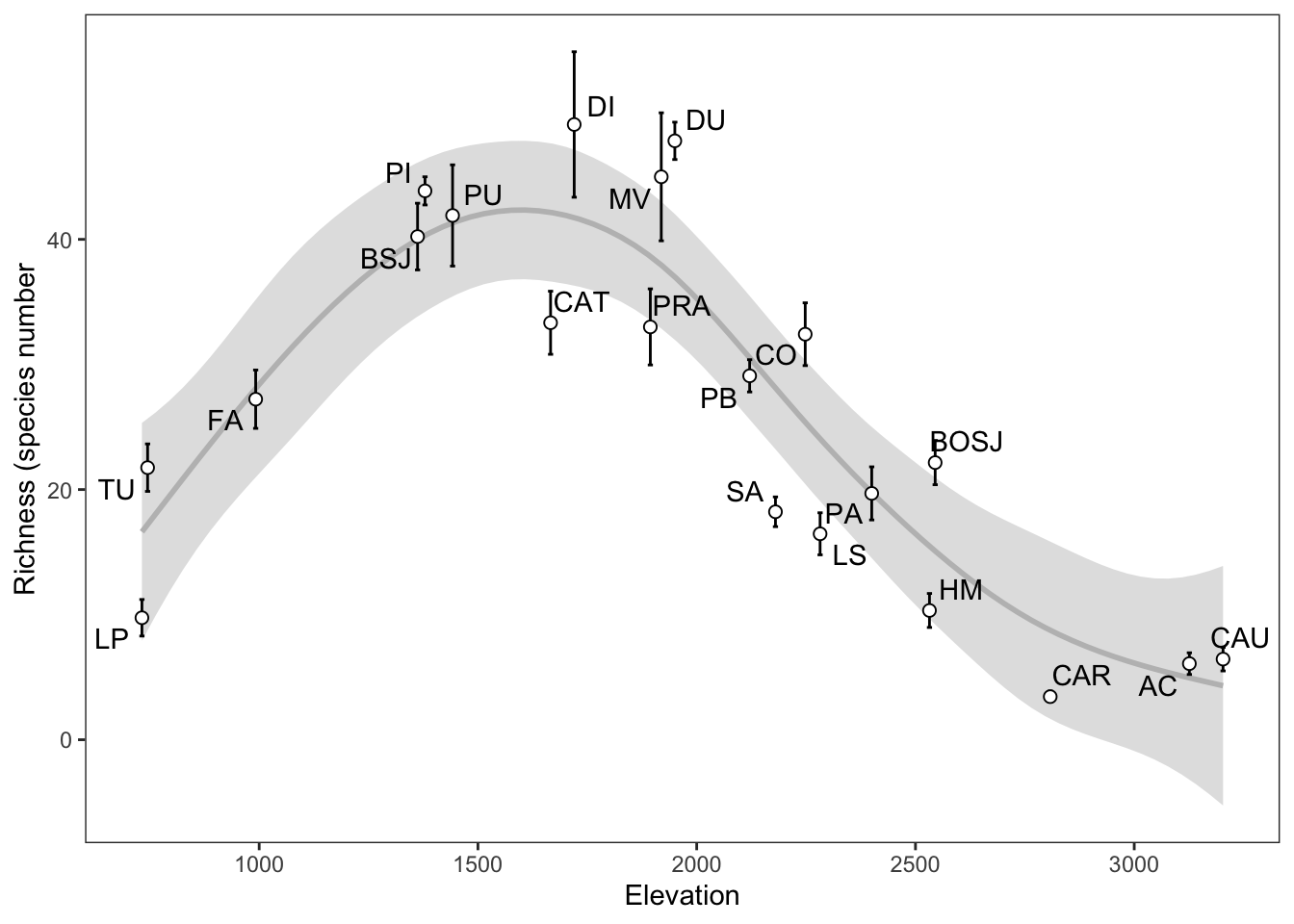Last updated: 2021-12-30

Checks: 7 0

Knit directory: ms_mariposas_biodiversity/

This reproducible R Markdown analysis was created with workflowr (version 1.7.0). The Checks tab describes the reproducibility checks that were applied when the results were created. The Past versions tab lists the development history.

Great! Since the R Markdown file has been committed to the Git repository, you know the exact version of the code that produced these results.

Great job! The global environment was empty. Objects defined in the global environment can affect the analysis in your R Markdown file in unknown ways. For reproduciblity it’s best to always run the code in an empty environment.

The command set.seed(20211228) was run prior to running the code in the R Markdown file. Setting a seed ensures that any results that rely on randomness, e.g. subsampling or permutations, are reproducible.

Great job! Recording the operating system, R version, and package versions is critical for reproducibility.

Nice! There were no cached chunks for this analysis, so you can be confident that you successfully produced the results during this run.

Great job! Using relative paths to the files within your workflowr project makes it easier to run your code on other machines.

Great! You are using Git for version control. Tracking code development and connecting the code version to the results is critical for reproducibility.

The results in this page were generated with repository version 2049a9a. See the Past versions tab to see a history of the changes made to the R Markdown and HTML files.

Note that you need to be careful to ensure that all relevant files for the analysis have been committed to Git prior to generating the results (you can use wflow_publish or wflow_git_commit). workflowr only checks the R Markdown file, but you know if there are other scripts or data files that it depends on. Below is the status of the Git repository when the results were generated:


Ignored files:
Ignored:    .Rhistory
Ignored:    .Rproj.user/

Untracked files:
Untracked:  analysis/prepara_datos_mariposas_all_years.Rmd

Unstaged changes:
Modified:   figs/plot_variables_elevation_allyears.pdf



Note that any generated files, e.g. HTML, png, CSS, etc., are not included in this status report because it is ok for generated content to have uncommitted changes.

These are the previous versions of the repository in which changes were made to the R Markdown (analysis/plot_variables_elevation_all_years.Rmd) and HTML (docs/plot_variables_elevation_all_years.html) files. If you’ve configured a remote Git repository (see ?wflow_git_remote), click on the hyperlinks in the table below to view the files as they were in that past version.

File Version Author Date Message
html 33b4560 ajpelu 2021-12-30 Build site.
html 381293c ajpelu 2021-12-30 Build site.
Rmd ce195a2 ajpelu 2021-12-30 nueva peticion de JMBA. Variables para todo el periodo

## Objetivo

• Crear un plot de variación de los parámetros con la elevación
library(tidyverse)
library(here)
library(ggpubr)
library(ggrepel)
denraw <- read_csv(here::here("data/densidad_by_year_alldata.csv"))
riqraw <- read_csv(here::here("data/riqueza_by_year_alldata.csv"))

densidad_avg <- denraw %>%
group_by(transecto, site, elev) %>%
summarise(mean = mean(den, na.rm = TRUE),
sd = sd(den, na.rm = TRUE),
se = sd/sqrt(n()))

ggplot(aes(x=elev, y = mean, label=site)) +
geom_smooth(method = "gam", formula = y ~ s(x, bs = "cs"), colour="gray", alpha=.3) +
# geom_smooth(method="loess", span=.5) +
geom_errorbar(aes(ymin = mean - se, ymax = mean + se)) +
geom_point(size=2, shape=21, fill="white") +
theme_bw() +
theme(
panel.grid = element_blank()
) +
ylab("Abundance (n ind / 100 m)") +
xlab("Elevation") +
geom_text_repel()

plot_densityVersion Author Date
381293c ajpelu 2021-12-30

diversidad_avg <- divraw %>%
group_by(transecto, site, elev) %>%
summarise(mean = mean(diversidad, na.rm = TRUE),
sd = sd(diversidad, na.rm = TRUE),
se = sd/sqrt(n()))

ggplot(aes(x=elev, y = mean, label = site)) +
geom_smooth(method = "gam", formula = y ~ s(x, bs = "cs"), colour="gray", alpha=.3) +
# geom_smooth() +
geom_errorbar(aes(ymin = mean - se, ymax = mean + se)) +
geom_point(size=2, shape=21, fill="white") +
theme_bw() +
theme(
panel.grid = element_blank()
) +
ylab("Diversity (Shannon index)") +
xlab("Elevation") +
geom_text_repel()

plot_diversityVersion Author Date
381293c ajpelu 2021-12-30

### Plot Riqueza

richness_avg <- riqraw %>%
group_by(transecto, site, elev) %>%
summarise(mean = mean(riq, na.rm = TRUE),
sd = sd(riq, na.rm = TRUE),
se = sd/sqrt(n()))

plot_richness <- richness_avg %>%
ggplot(aes(x=elev, y = mean, label = site)) +
geom_smooth(method = "gam", formula = y ~ s(x, bs = "cs"), colour="gray", alpha=.3) +
# geom_smooth() +
geom_errorbar(aes(ymin = mean - se, ymax = mean + se)) +
geom_point(size=2, shape=21, fill="white") +
theme_bw() +
theme(
panel.grid = element_blank()
) +
ylab("Richness (species number") +
xlab("Elevation") +
geom_text_repel()

plot_richnessVersion Author Date
381293c ajpelu 2021-12-30

### Todas las variables juntas

p <- bind_rows(
richness_avg %>% mutate(variable = "riq"),

mylabel <- c(
den = "Abundance (n ind / 100 m)",
div = "Diversity (Shannon index)",
riq = "Richness (species number)")

plot_variables <- p %>%
ggplot(aes(x=elev, y = mean, label = site)) +
geom_smooth(method = "gam", formula = y ~ s(x, bs = "cs"), colour="blue", alpha=.1, fill="blue") +
# geom_smooth() +
geom_errorbar(aes(ymin = mean - se, ymax = mean + se)) +
geom_point(size=2, shape=21, fill="blue") +
theme_bw() +
theme(
panel.grid = element_blank(),
strip.text = element_text(face = "bold"),
strip.background = element_rect(fill="white")
) + geom_text_repel() +
facet_wrap(~variable, ncol=1, scales = "free_y",
labeller = labeller(variable = mylabel),
strip.position="top") +
xlab("Elevation") + ylab("") 
ggsave(here::here("figs/plot_variables_elevation_allyears.pdf"),
device = "pdf",
width = 5, height = 7.5)
plot_variablesVersion Author Date
381293c ajpelu 2021-12-30
dev.off()
null device
1 
ggsave(plot= plot_variables,
here::here("figs/plot_variables_elevation_allyears.png"),
device = "png",
dpi = 300,
width = 5, height = 7.5)

sessionInfo()
R version 4.0.2 (2020-06-22)
Platform: x86_64-apple-darwin17.0 (64-bit)
Running under: macOS Catalina 10.15.3

Matrix products: default
BLAS:   /Library/Frameworks/R.framework/Versions/4.0/Resources/lib/libRblas.dylib
LAPACK: /Library/Frameworks/R.framework/Versions/4.0/Resources/lib/libRlapack.dylib

locale:
 en_US.UTF-8/en_US.UTF-8/en_US.UTF-8/C/en_US.UTF-8/en_US.UTF-8

attached base packages:
 stats     graphics  grDevices utils     datasets  methods   base

other attached packages:
 ggrepel_0.9.1   ggpubr_0.4.0    here_1.0.1      forcats_0.5.1
 tidyr_1.1.3     tibble_3.1.2    ggplot2_3.3.5   tidyverse_1.3.1
 workflowr_1.7.0

loaded via a namespace (and not attached):
 nlme_3.1-152      fs_1.5.0          lubridate_1.7.10  httr_1.4.2
 rprojroot_2.0.2   tools_4.0.2       backports_1.2.1   bslib_0.2.4
 utf8_1.1.4        R6_2.5.1          DBI_1.1.1         mgcv_1.8-33
 colorspace_2.0-2  withr_2.4.1       tidyselect_1.1.1  processx_3.5.1
 curl_4.3          compiler_4.0.2    git2r_0.28.0      textshaping_0.3.2
 cli_2.5.0         rvest_1.0.0       xml2_1.3.2        labeling_0.4.2
 sass_0.3.1        scales_1.1.1.9000 callr_3.7.0       systemfonts_1.0.0
 digest_0.6.27     foreign_0.8-81    rmarkdown_2.8     rio_0.5.16
 pkgconfig_2.0.3   htmltools_0.5.2   highr_0.8         dbplyr_2.1.1
 ragg_1.1.1        ellipsis_0.3.2